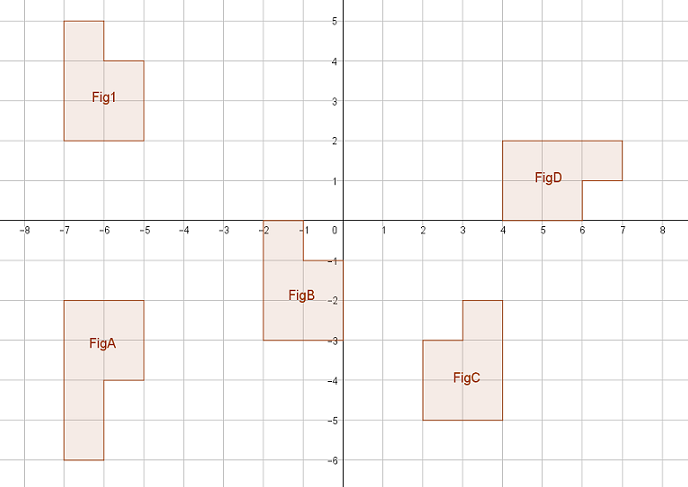Match Fishtank is now Fishtank Learning!

# Transformations and Angle Relationships

## Objective

Review rigid transformations and congruence between two figures.

## Common Core Standards

### Core Standards

?

• 8.G.A.1.A — Lines are taken to lines, and line segments to line segments of the same length.

• 8.G.A.1.B — Angles are taken to angles of the same measure.

• 8.G.A.1.C — Parallel lines are taken to parallel lines.

• 8.G.A.2 — Understand that a two-dimensional figure is congruent to another if the second can be obtained from the first by a sequence of rotations, reflections, and translations; given two congruent figures, describe a sequence that exhibits the congruence between them.

• 8.G.A.3 — Describe the effect of dilations, translations, rotations, and reflections on two-dimensional figures using coordinates.

## Criteria for Success

?

1. Understand the properties of rigid transformations and their impact on lines, line segments, angle measures, and parallel line relationships.
2. Describe a sequence of transformations that demonstrates congruency between two figures.
3. Perform a sequence of transformations to map one figure to another.
4. Describe the effects of transformations using coordinate points.

## Tips for Teachers

?

This can be used as a flex day, depending on the needs of your students. As a result, there are no Anchor Problems. The Problem Set Guidance includes resources, activities, and problem ideas that can be used as a whole class or in small group activities.

## Problem Set

?The following resources include problems and activities aligned to the objective of the lesson that can be used to create your own problem set.

• Activity: Students create their own transformation sequences on graph paper and then swap with another student who describes/writes the sequence, and vice versa
• Khan Academy Geometric TransformationsThis explores how virtual animation sets are created using geometric transformations. It is a great way to show students how transformations are used in digital arts and give them experience working with transformations online (MP.4). Requires computers.
• MARS Summative Assessment Tasks for Middle School Aaron's DesignsAfter this problem, students could create their own designs using transformations
• Illustrative Mathematics Point Reflection
• This is a fantastic activity that allows students to explore transformations using technology; highly recommended. Computers are required.
• MARS Formative Assessment Lesson for Grade 8 Representing and Combining TransformationsA maze-like activity where students draw connections to different images using transformations. This includes reflections over the lines y=x and y=-x. These can be removed, or students may need additional guidance since these weren't covered in lessons
• Illustrative Mathematics Reflecting a Rectangle over a DiagonalChallenge

?

Figure 1 is shown in the coordinate plane below. Four other figures are shown: Figures ${A, B, C,}$ and $D$1. Which of the four figures are congruent to Figure 1?
2. For two of those figures identified in part (a), describe a sequence that proves the congruency between Figure 1 and the other figure.

?